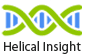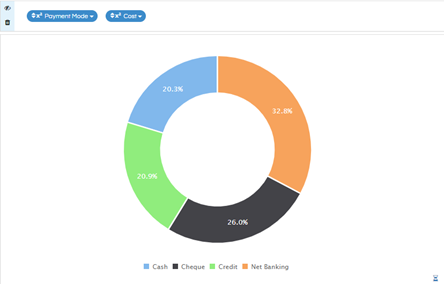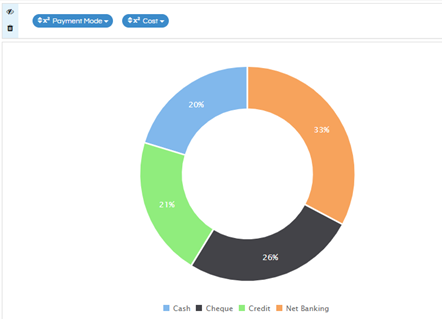# Round off Percent Label in Donut Chart

Hello Helical Insight,

I am using helical insight 4.0 EE. I have a donut chart in which the labels are showing as percentage. I want to remove the decimal values and round off to the nearest whole value. I have tried applying round function to the column, but that does not work.Thanks,
Netta.

Hello Netta,

If you apply round function to the column, the actual value gets rounded. However, since the label is displayed as percentage of total, it will still have the decimal values.

In order to remove the decimal and round off to nearest whole number, you can apply the following script in the JS editor. This below code will also work on the donut chart also:

``````hi_container.set("preExecution", function(a,b) {
console.log(a);
var b = a.get("viz_Options");
b.chartOptions.donut = {
label: {
show: true,
format: function (value, ratio, id) {
return Math.round(ratio*100)+'%';
}
}
};
a.set("viz_Options", b);
});
``````Thanks,
Helical Insight